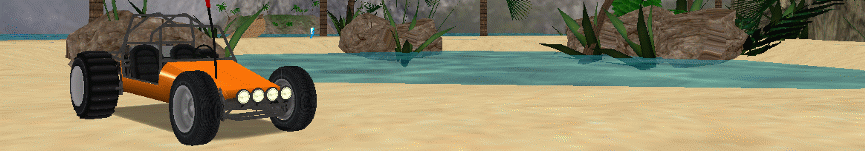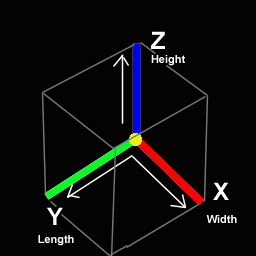Re-Volt I/O

# Car Body Details

Edit
Date: January 9, 2017
Authors: Gel38
Categories: Parameters
Tags: car_making``````;====================
; Car Body details
;====================

BODY { ; Start Body
ModelNum 0
Offset 0, 0, 0
Mass 1.400000
Inertia 780.000000 0.000000 0.000000
0.000000 1015.000000 0.000000
0.000000 0.000000 445.000000
Gravity 2200
Hardness 0.000000
Resistance 0.001000
AngRes 0.001000
ResMod 25.000000
Grip 0.010000
StaticFriction 0.800000
KineticFriction 0.400000
} ; End Body``````

### 1. ModelNum

This corresponds with the "Model Filenames" section. This setting uses the numbers -1 to 18 and determines which model is used. If no models are used, this number should be set to -1, otherwise from 0 to 18. In this case you want the number corresponding with body.prm.

### 2. Offset

These numbers determine the placement of your 3d model. It uses an XYZ coordinate system.`X-0, Z-0, Y-0`

X = width of car (This is the 1st number) Y = length of car (The 3rd number) Z = height of car(2nd number)

Positive numbers on the X axis place the model to the Right, whereas, Negative numbers place it to the Left.

Positive numbers on the Z axis will move the model down toward the ground, whereas, Negative numbers will bring it up.

Positive numbers on the Y axis move the model forward, whereas, Negative numbers it to the rear

If you move your car too far upwards, and it flips, the car will go under the ground, as this setting does not move your collision hull.hul file.

### 3. Mass

This is the weight of the body of your car in kilograms (kg). This setting will affect your speed and acceleration as well.

Lighter car = more speed and acceleration Heavier car = less speed and slower acceleration.

### 4. Inertia

This setting tells Re-Volt exactly how big your car is. It works on a cubed XYZ coordinate system. It looks something like this

Vertical Axis | Linear Axis X X, Y, Z Y X, Y, Z Z X, Y, Z

On the 1st line, only the X axis (1st number) should be filled in. On the 2nd line, only the Y axis (2nd number) should be filled in. On the 3rd line, only the Z axis (3rd number) should be filled in.

How to calculate the Inertia numbers:

Use Positive numbers when doing these formulas.

X line: Add the distance between your Left (Represented by L) and Right (R) tires in RV units, preferably the widest distance, then multiply by 25. Inertia will be represented by N. Here are 2 formula options for this number:

(R + L) 25 = N or R 2 * 25 = N

Y line: Add the distance between your Front wheel (F) and Rear Wheel (R) multiplied by 25.

(F+R) * 25 = N

Z line: This is the height of your car (H) multiplied by 25.

H * 25 = N

A longer car will turn more sluggishly. A wide car will be less likely to roll over and may have stiff handling. A tall car will be more likely to roll over and may be tossed about more easily.

### 5. Gravity

This determines the amount of time it takes the car to catch grip when it lands after a jump (thus, squealing tires). The lower the number, the more time it takes to catch grip. A value of 100 takes about 5 seconds to catch grip.

### 6. Hardness

This is how hard the shell of your car is. Higher numbers make the car bouncy, alot like a fully inflated beachball. Extremely high numbers make the car uncontrollable. Low numbers cause the car to take light impacts very well, but the car will bounce after a hard hit like a rebounding rubber ball.

### 7. Resistance

This, along with the following 2 settings deal with the aerodynamics of your car. Resistance deals with air resistance while driving (drag). High numbers will increase the drag on your car and reduce your top speed. Low numbers (in excess of .0001) reduces the drag created and greatly increase your top speed. This setting will not affect the rotation of the car.

### 8. AngRes

This is the rotational air resistance (aptitude to turn end over end during a jump). A value which is higher than .001 will cause the car to rotate less quickly in the air, and extremely high numbers will stop rotation in the air (very unrealistic). Lower numbers can cause the car to rotate more quickly in the air. For cars with more weight in the front of the car (CoM is in a forward position) a lower number is good. Negative numbers are not reccommended as, the car will spin wildly out of control while in the air.

### 9. ResMod

This is the angular air resistance scale when airborne (or in-air stability). Numbers around the Re-Volt default of 25 to 30 (and higher) will cause the car to be more stable in the air. However, numbers which are too high will stop the rotation of the car. Lower values will cause the car to act more realistically as if the car weighs more on the right or left side. This makes the car more erratic. This seems to have an effect on the overall handling of the car when in the air.

### 10. Grip

This is the general grip of the car body. Higher values will cause it to stick to the track better, like a magnet. Lower values will decrease this friction.

### 11. StaticFriction

This is the car body's resistance to movement while not moving. If the value is high, and the car is upside-down, not moving, then is hit by another car. It will not be inclined to move so much. If the number is lower, the car will be more prone to being moved. This also controls how much the body sticks to and opposes movement when touching a wall. It is not recommended to set the value to 0, and should always be set higher than kinetic friction.

### 12. KineticFriction

This is the car body's resistance to movement while moving. If the value is high, and the car is upside-down, moving, and then is hit by another car, it will not be inclined to move much father. If the number is lower, the car will be more prone to being moved with ease. This also controls how much the car will slow down when the body sticks to a wall. It is not recommended to set the value to 0.